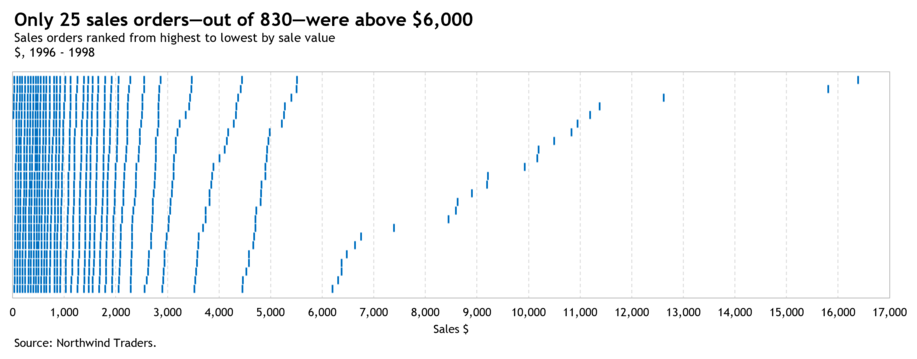• WORK
• TOOLS
• TRAINING
• VIEWS
• CONTACT

Views > Excel charts > How to create the zvinca plot in Excel

One common use of the horizontal bar chart is to rank a list of items based on their values. Very often the question in business is: how things rank. For example: Who are my top customers. Who are my least performing salespeople. What products are selling the most.

Bar graphs can be effective at ranking items up to a limit—the size of the screen or the size of the paper. So how do we rank 830 items? The bar chart below does a good job at ranking 830 sales orders by the value of the sale while at the same time showing the shape of the data. However, it's difficult to see the individual values.

15. Select the range I1:J831 and then click the Insert tab. In the Charts group click the Insert Scatter (X,Y) or Bubble Chart icon and pick the Scatter chart. The chart should look like this:

# HOW TO CREATE THE ZVINCA PLOT IN EXCEL

HOW TO CREATE THE ZVINCA PLOT IN EXCEL

Four years later, in 2017, Stephen and Daniel Zvinca collaborated together and came up with a new solution they called the (I highly recommend to follow Daniel on Twitter. He is a man of few tweets, but when he does tweet, he's laser sharp and to the point).

Similar to the Wrapped Bar Graph, the Zvinca Plot splits the values into subsets. Where the two chart types differ, however, is that in the Zvinca Plot those subsets are displayed relative to a single quantitative scale rather than several scales as done in the Wrapped Bar Graph.

Here below you see the same 830 sales orders displayed using the Zvinca Plot—with a subset size of 50. Lets build it in Excel.

The data for the above chart is from Microsoft's classic relational Northwind database as shown in the screenshot below:

1. Select a cell within the data.
2. Press Ctrl + T to open the Create Table dialog box. Press the Ok button to close the Create Table dialog box.
3. In the Properties group, under the Table Design tab, type a new table name (i.e. tblData) in the Table Name box and press Enter.
4. Next, press the filter button in the "Sales" header row and pick the Sort Largest to Smallest sort order from the menu. The data should look like the snapshot below:

5. Enter the labels "Count", "Subset size" and "Step" in cells L1, L2 and L3 respectively.
6. Enter the following formula in cell M1:
= COUNTA(tblData[Order ID])
7. Enter the number 50 in cell M2.
8. Enter the following formula in cell M3:
= 1 / (M2 + 1)

Because I'm using a subset size of 50, I need to generate a sequence of 52 numbers starting at 0. That sequence would help in generating y coordinates between 0 and 1 with an equal spacing of 1 / 51 = 0.019608 as follows:

9. Enter the following formula in cell D2:
= SEQUENCE(M2 + 2,1,0)
10. Enter the following formula in cell E2:
= M3 * (M2 + 1 - D2#)

Next, I need to create a scatterplot to display the data. For that I need to assign the right y coordinate to each sale order. This should be done after subdividing the sales orders into subsets of 50 data points each as follows:

11. Enter the following formula in cell G2:
= SEQUENCE(M1,1)
12. Enter the following formula in cell H2:
=IF(MOD(G2#,M2)=0,M2,MOD(G2#,M2))
13. Enter the following formula in cell I2:
= tblData[Sales]
14. Enter the following formula in cell J2:
= INDEX(E2#,MATCH(H2#,D2#,0))

Like that I've prepared the data setup of x and y coordinates required to build the scatterplot as per the screenshot below:

To solve this problem, Stephen Few came up in 2013 with the Wrapped Bar Graph to extend the number of values that can be displayed in a bar graph. He explained his new invention in his paper Wrapping Graphs to Extend Their Limits.

As shown in the graph below—taken from Few's paper—the idea is to split the data into multiple columns, sort the bars in order from largest to smallest and from left to right, and limit the quantitative scale associated with each column to the range that’s needed for the values in that column.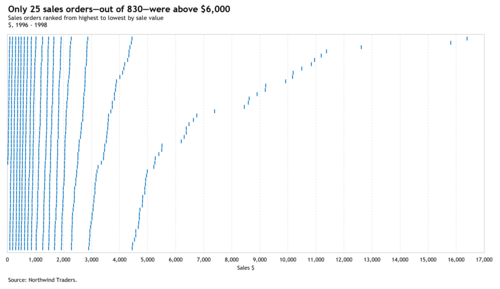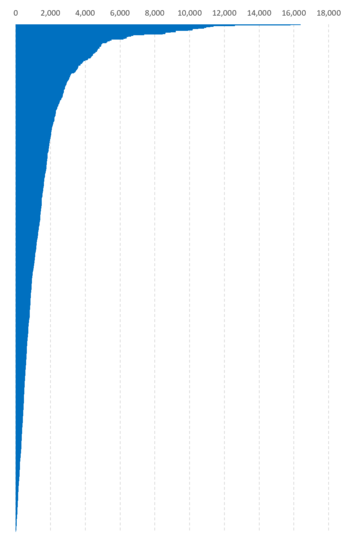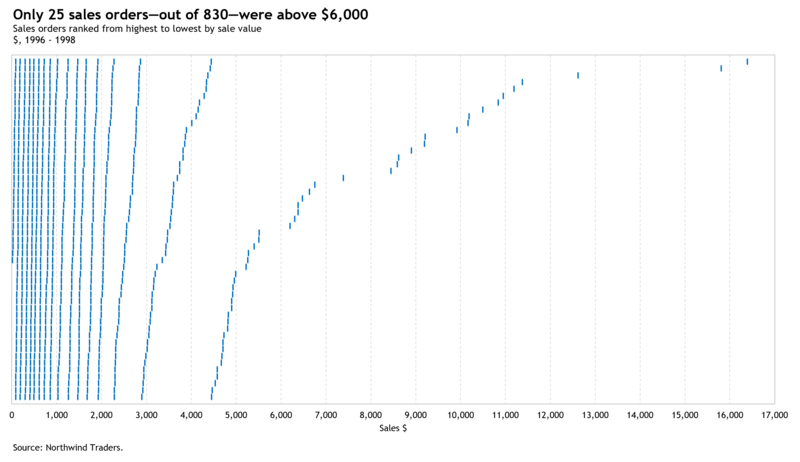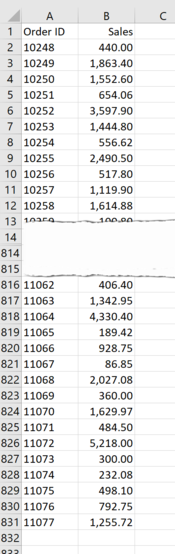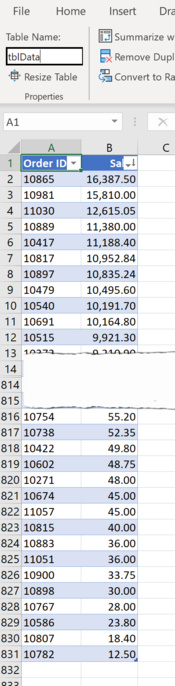Thank you for reading, and I hope you found this useful. If you have any questions, find me on Twitter and ask me anything.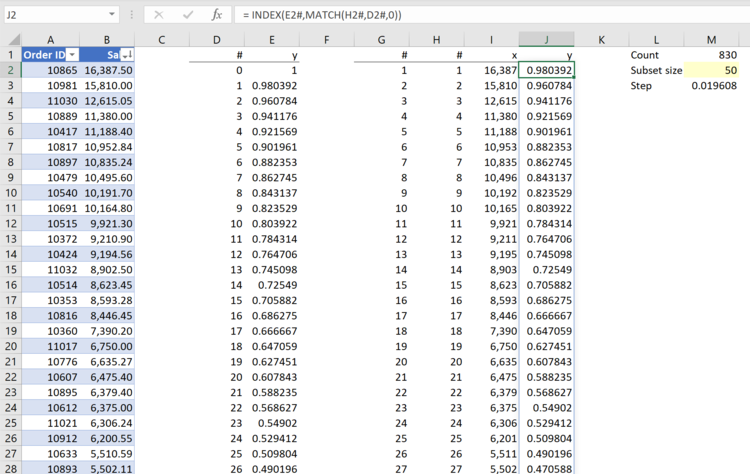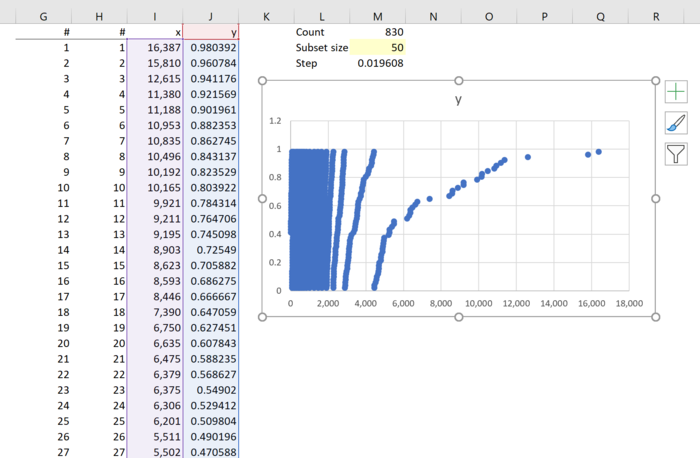16. Constrain the vertical axis Bounds Maximum to 1.
17. Constrain the horizontal axis Bounds Maximum to 17,000 and its Units Major to 1,000.
18. Delete the chart title, the vertical axis and the horizontal grids.
19. Add a horizontal axis title. Turn off the border of the Chart Area by setting the border to No line
. Format the border of the Plot Area to Solid line with grey color. Change the Dash type of the vertical gridlines to Dash. The chart should look like this: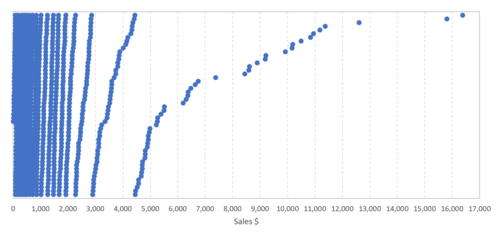20. Draw a short vertical line anywhere on the worksheet using the Shapes in the Illustration Group under the Insert tab.
21. Copy the newly created shape to the Clipboard by pressing Ctrl + C.
22. Select the markers of the data series in the chart and then paste the shape by pressing Ctrl + V.
23. Add a chart title and source. Resize the chart and the chart will look like this: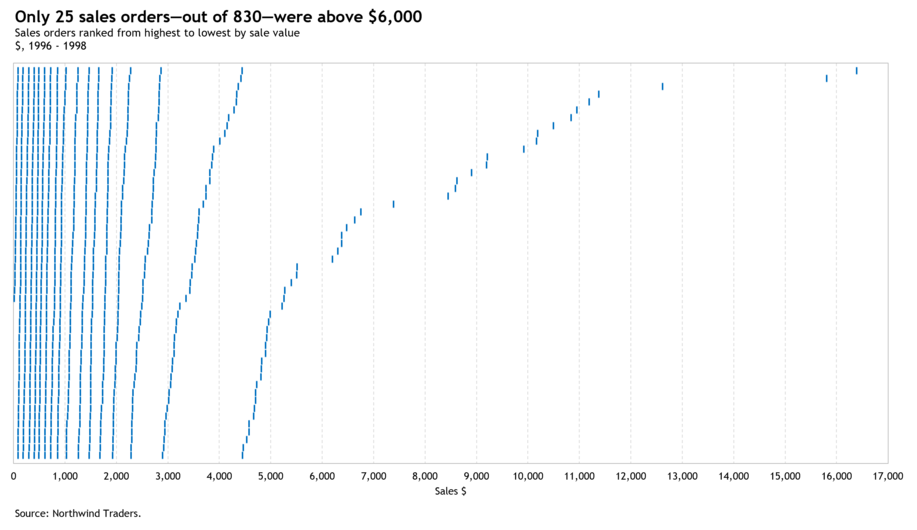24. Try another Subset size, say 25, and the chart will look like this: